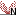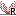# Statistical analysis - identifying differential expression

The CLC Genomics Workbench is designed to help you identify differential expression. You have a choice of a number of standard statistical tests, that are suitable for different data types and different types of experimental settings. There are two main types of tests: tests that assume that data consists of counts and compare these or their proportions (described in Empirical analysis of DGE and Tests on proportions) and tests that assume that the data is real-valued, has Gaussian distributions and compare means (described in Gaussion tests). To run the statistical analysis:

Toolbox | Transcriptomics Analysis ()| Statistical Analysis | Empirical Analysis of DGE ()

Toolbox | Transcriptomics Analysis ()| Statistical Analysis | On Proportions ()

or  Toolbox | Transcriptomics Analysis ()| Statistical Analysis | On Gaussian Data ()

For all kinds of statistical analysis you first select the experiment () that you wish to use and click Next (learn more about setting up experiments).

The first part of the explanation of how to proceed and perform the statistical analysis is divided into three, depending on whether you are doing Empirical analysis of DGE, tests on proportions or Gaussian-based tests. The last part has an explanation of the options regarding corrected p-values which applies to all tests.

Subsections